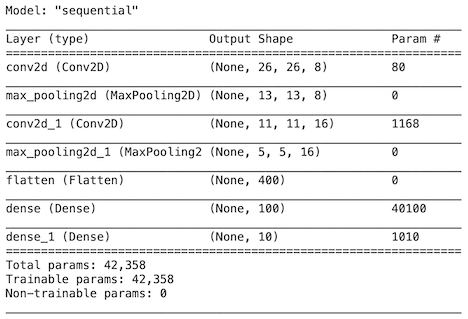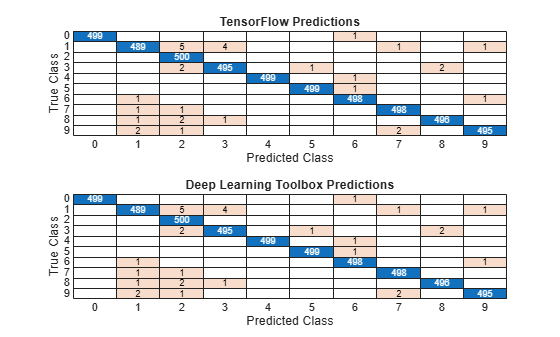# Inference Comparison Between TensorFlow and Imported Networks for Image Classification

This example shows how to compare the inference (prediction) results of a TensorFlow™ network and the imported network in MATLAB® for an image classification task. First, use the network for prediction in TensorFlow and save the prediction results. Then, import the network in MATLAB using the `importTensorFlowNetwork` function and predict the classification outputs for the same images used to predict in TensorFlow.

This example provides the supporting files `digitsNet.zip` and `TFData.mat`. To access these supporting files, open the example in Live Editor.

### Image Data Set

Load the Digits data set. The data contains images of digits and the corresponding labels.

`[XTest,YTest] = digitTest4DArrayData;`

Create the test data that the TensorFlow network uses for prediction. Permute the 2-D image data from the Deep Learning Toolbox™ ordering (`HWCN`) to the TensorFlow ordering (`NHWC`), where `H`, `W`, and `C` are the height, width, and number of channels of the images, respectively, and `N` is the number of images.

```x_test = permute(XTest,[4,1,2,3]); y_test = double(string(YTest));```

Save the data to a MAT file.

```filename = "digitsMAT.mat"; save(filename,"x_test","y_test")```

### Inference with Pretrained Network in TensorFlow

Load a pretrained TensorFlow network for image classification in Python® and classify new images.

Import libraries.

```import tensorflow as tf import scipy.io as sio ```

Load the test data set from `digitsMAT.mat`.

```data = sio.loadmat("digitsMAT.mat") x_test = data["x_test"] y_test = data["y_test"] ```

Load the `digitsNet` pretrained TensorFlow model, which is in the saved model format. If the folder is archived in `digitsNet.zip`, extract the archived contents of `digitsNet.zip` into the current folder.

```from tensorflow import keras model = keras.models.load_model("digitsNet") ```

Display a summary of the model.

```model.summary() ```Classify new digit images.

```scores = model.predict(tf.expand_dims(x_test,-1)) ```

Save the classification scores in the MAT file `TFData.mat`.

```sio.savemat("TFData.mat", {"scores_tf":scores}) ```

### Inference with Imported Network in MATLAB

Import the pretrained TensorFlow network into MATLAB using `importTensorFlowNetwork` and classify the same images as in TensorFlow.

Specify the model folder, which contains the `digitsNet` TensorFlow model in the saved model format.

```if ~exist("digitsNet","dir") unzip("digitsNet.zip") end modelFolder = "./digitsNet";```

Specify the class names.

`classNames = string(0:9);`

Import the TensorFlow network in the saved model format. By default, `importTensorFlowNetwork` imports the network as a `DAGNetwork` object.

`net = importTensorFlowNetwork(modelFolder,Classes=classNames);`
```Importing the saved model... Translating the model, this may take a few minutes... Finished translation. Assembling network... Import finished. ```

Display the network layers.

`net.Layers`
```ans = 13x1 Layer array with layers: 1 'conv2d_input' Image Input 28x28x1 images 2 'conv2d' 2-D Convolution 8 3x3x1 convolutions with stride [1 1] and padding [0 0 0 0] 3 'conv2d_relu' ReLU ReLU 4 'max_pooling2d' 2-D Max Pooling 2x2 max pooling with stride [2 2] and padding [0 0 0 0] 5 'conv2d_1' 2-D Convolution 16 3x3x8 convolutions with stride [1 1] and padding [0 0 0 0] 6 'conv2d_1_relu' ReLU ReLU 7 'max_pooling2d_1' 2-D Max Pooling 2x2 max pooling with stride [2 2] and padding [0 0 0 0] 8 'flatten' Keras Flatten Flatten activations into 1-D assuming C-style (row-major) order 9 'dense' Fully Connected 100 fully connected layer 10 'dense_relu' ReLU ReLU 11 'dense_1' Fully Connected 10 fully connected layer 12 'dense_1_softmax' Softmax softmax 13 'ClassificationLayer_dense_1' Classification Output crossentropyex with '0' and 9 other classes ```

Predict class labels and classification scores using the imported network.

`[labels_dlt,scores_dlt] = classify(net,XTest);`

For this example, the data `XTest` is in the correct ordering. Note that if the image data `XTest` is in TensorFlow dimension ordering, you must convert `XTest` to the Deep Learning Toolbox ordering by entering `Xtest = permute(Xtest,[2 3 4 1])`.

### Compare Accuracy

Load the TensorFlow network scores from `TFData.mat`.

`load("TFData.mat")`

Compare the inference results (classification scores) of the TensorFlow network and the imported network.

`diff = max(abs(scores_dlt-scores_tf),[],"all")`
```diff = single 5.3048e-06 ```

The difference between inference results is negligible, which strongly indicates that the TensorFlow network and the imported network are the same.

As a secondary check, you can compare the classification labels. First, compute the class labels predicted by the TensorFlow network. Then, compare the labels predicted by the TensorFlow network and the imported network.

```[~,ind] = max(scores_tf,[],2); labels_tf = categorical(classNames(ind))'; isequal(labels_dlt,labels_tf)```
```ans = logical 1 ```

The labels are the same, which indicates that the two networks are the same.

Plot confusion matrix charts for the labels predicted by the TensorFlow network and the imported network.

```tiledlayout(2,1) nexttile confusionchart(YTest,labels_tf) title("TensorFlow Predictions") nexttile confusionchart(YTest,labels_dlt) title("Deep Learning Toolbox Predictions")```